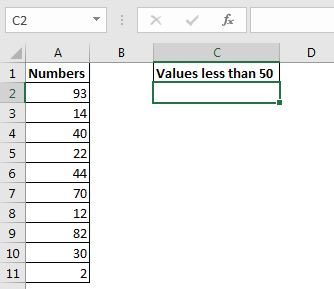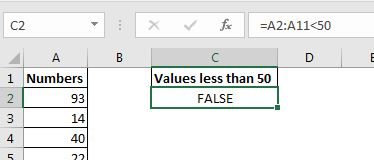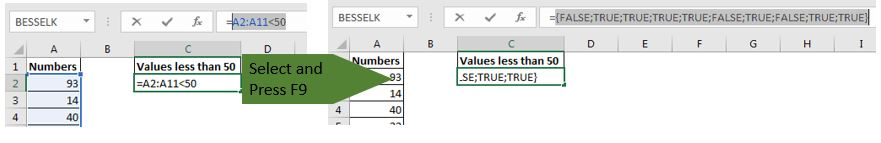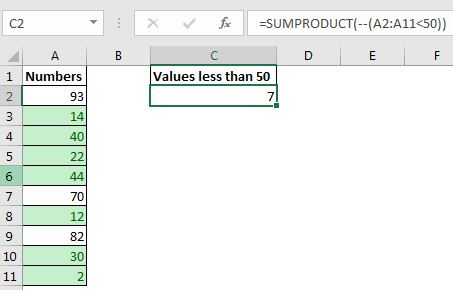# The Double Negatives (--) in Excel

You must have seen these strange -- (double negative) signs in some advance formulas. If you try removing them from the formula, the formula stops working. You must be wondering what exactly are these double minus sign doing in excel formula?

Double negatives (--) convert boolean values into binary values.

These double negative (--) signs are used to convert TRUE and FALSE values into 1s and 0s. Mostly used with array function and formulas of excel. Let's see an example.

## Excel -- Double Negative ExampleHere I have some numbers. I want to count the numbers that are less than 50. I know that it can be done easily using the COUNTIF function but since we want to learn about the double minus symbol in excel formulas, we will use the SUMPRODUCT function to do this.

 =A2:A11<50If you hit enter, it will show FALSE. But under the hood, it is returning an array of TRUE and FALSE. You can check it by selecting the part of formula in edit mode and then by pressing the F9 key. {FALSE;TRUE;TRUE;TRUE;TRUE;FALSE;TRUE;FALSE;TRUE;TRUE}. TRUE for each value that is less than 50 in range A2:A11.To convert these boolean values into 1s and 0s, put -- sign before this statement.

 =--(A2:A11<50)

When you hit enter, it will show 0. Since the first value is not less than 50. But when you use debugging key F9, you will see an array of 1s and 0s. {0;1;1;1;1;0;1;0;1;1}. 1 for each TRUE value.

Now, if we sum this array up, we will get the number of values that are less than 50.

To do so, let's wrap this formula into the SUMPRODUCT function.

 =SUMPRODUCT(--(A2:A11<50))

This formula will sum up the array returned by the internal statement. We get the answer 7.Try Removing -- from the formula

Now if you remove -- from this formula and make it =SUMPRODUCT((A2:A11<50)), it will return 0. Because the array returned by A2:A11<50 contains boolean values and SUMPRODUCT function can't sum boolean values.

### Why -- double minus sign only?

It is not necessary to use only double negative to convert boolean values into binary values.

You can add 0 to boolean values, multiply by 1, or use N function, to do the same as an alternative.

 =SUMPRODUCT((A2:A11<50)+0) =SUMPRODUCT((A2:A11<50)*1) =SUMPRODUCT(N(A2:A11<50))

All of the above formulas will achieve the task.

I used a simple scenario to explain the use -- sign in excel formulas. It was not needed in this task but there are the scenarios where you will need to convert boolean values into binary values. Below are some examples.

Related Articles: Examples of the use of double negative

Count total matches in two ranges in Excel | The SUMPRODUCT function along with double -- sign can easily count matching values in two ranges.

2 Ways to Sum by Month in Excel | With help minus sign we can sum values by month from a date. We will not need to extract month name from date.

Check if a string contains one of many texts | With the help of double negatives, we can check if a string contains any of the text from a given list.

Popular Articles:

Wildcards in Excel | The Wildcard characters are * and ? in excel. These are used with text related formulas, where the exact match is not guaranteed.

50 Excel Shortcuts to Increase Your Productivity | Get faster at your task. These 50 shortcuts will make you work even faster on Excel.

The VLOOKUP Function in Excel | This is one of the most used and popular functions of excel that is used to lookup value from different ranges and sheets.

COUNTIF in Excel 2016 | Count values with conditions using this amazing function. You don't need filter your data to count specific value. Countif function is essential to prepare your dashboard.

How to Use SUMIF Function in Excel | This is another dashboard essential function. This helps you sum up values on specific conditions.

Terms and Conditions of use

The applications/code on this site are distributed as is and without warranties or liability. In no event shall the owner of the copyrights, or the authors of the applications/code be liable for any loss of profit, any problems or any damage resulting from the use or evaluation of the applications/code.